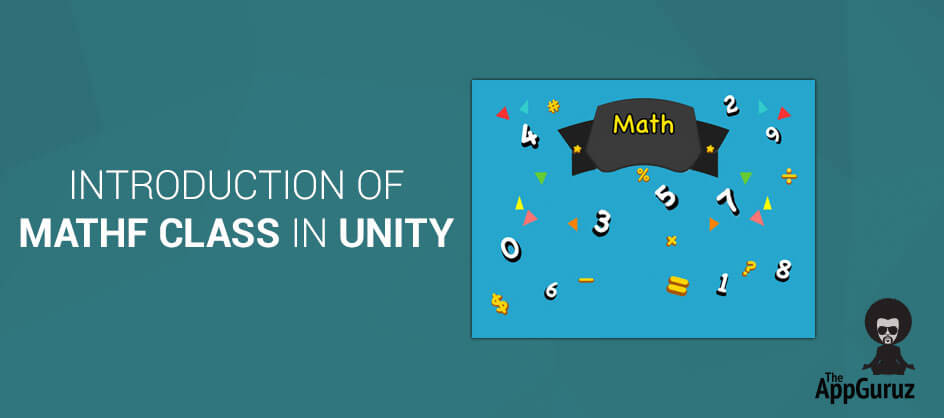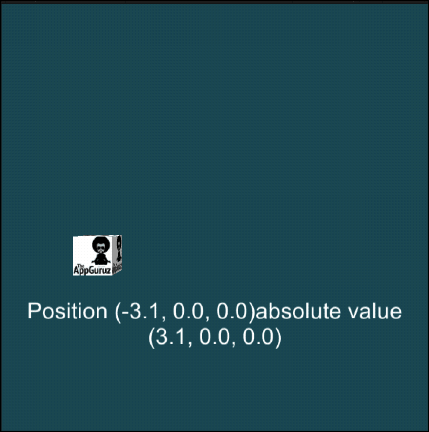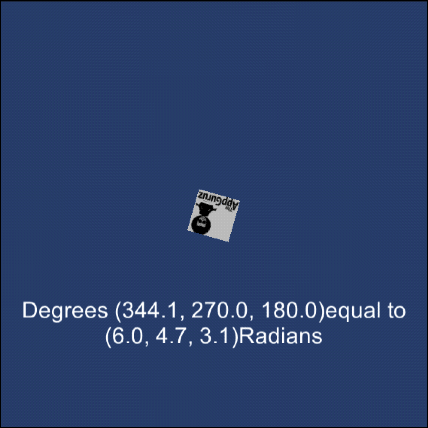## Objective

Main objective of this blog post is to give you an idea about Math F Class in Unity.

A Mathf class in unity is a collection of common math functions. Mathf provides some static functions and static variables listed as below:

## Step 1 Static Functions

 Abs Returns the absolute value of f. Acos Returns the arc-cosine of f - the angle in radians whose cosine is f. Approximately Compares two floating point values if they are similar. Asin Returns the arc-sine of f - the angle in radians whose sine is f. Atan Returns the arc-tangent of f - the angle in radians whose tangent is f. Atan2 Returns the angle in radians whose Tan is y/x. Ceil Returns the smallest integer greater to or equal to f. CeilToInt Returns the smallest integer greater to or equal to f. Clamp Clamps a value between a minimum float and maximum float value. Clamp01 Clamps value between 0 and 1 and returns value. ClosestPowerOfTwo Returns the closest power of two value. Cos Returns the cosine of angle f in radians. DeltaAngle Calculates the shortest difference between two given angles. Exp Returns e raised to the specified power. Floor Returns the largest integer smaller to or equal to f. FloorToInt Returns the largest integer smaller to or equal to f. GammaToLinearSpace Converts the given value from gamma to linear color space. InverseLerp Calculates the Lerp parameter between of two values. IsPowerOfTwo Returns true if the value is power of two. Lerp Interpolates between a and b by t. t is clamped between 0 and 1. LerpAngle Same as Lerp but makes sure the values interpolate correctly when they wrap around 360 degrees. LinearToGammaSpace Converts the given value from linear to gamma color space. Log Returns the logarithm of a specified number in a specified base. Log10 Returns the base 10 logarithm of a specified number. Max Returns largest of two or more values. Min Returns the smallest of two or more values. MoveTowards Moves a value current towards target. MoveTowardsAngle Same as MoveTowards but makes sure the values interpolate correctly when they wrap around 360 degrees. NextPowerOfTwo Returns the next power of two value. PerlinNoise Generate 2D Perlin noise. PingPong PingPongs the value t, so that it is never larger than length and never smaller than 0. Pow Returns f raised to power p. Repeat Loops the value t, so that it is never larger than length and never smaller than 0. Round Returns f rounded to the nearest integer. RoundToInt Returns f rounded to the nearest integer. Sign Returns the sign of f. Sin Returns the sine of angle f in radians. SmoothDamp Gradually changes a value towards a desired goal over time. SmoothDampAngle Gradually changes an angle given in degrees towards a desired goal angle over time. SmoothStep Interpolates between min and max with smoothing at the limits. Sqrt Returns square root of f. Tan Returns the tangent of angle f in radians.

## Step 3 Implementation

### 3.1 Mathf Function

Here, I’ll explain some of the functions of Mathf class. So let's look at the functions that we are going to use in this example.

#### 3.1.1 Abs

Syntax and Description of Abs is as under:

 Syntax public static float Abs (float f); Description Returns the absolute value of f. An absolute value is not negative. It is same as the first value without the negative sign. So Mathf.Abs(-3.1f) function will return 3.1.

#### 3.1.2 Acos

Syntax and Description of Acos is as under:

 Syntax public static float Acos(float f); Description Returns the arc-cosine of f. Arc-cosine of f is the angle in radians whose cosine is f. So Mathf.Acos(0.5f) will return PI/3 i.e. 1.047198.

#### 3.1.3 Atan

Syntax and Description of Atan is as under:

 Syntax public static float Atan2 (float y, float x); Description Returns the angle in radians whose value is y/x. This function returns the angle between the x-axis and a 2D vector starting at zero and terminating at (x, y). So the function will not throw a division by zero exception even if the value of x is zero.

#### 3.1.4 Deg2Red

Syntax and Description of Deg2Red is as under:

 Syntax public static float Deg2Rad; Description It is a static constant that is used for converting angle from degree to its according radian value. It has a constant value equal to (PI *2)/360.

### 3.2 Scripting

Let’s create one short demo of these functions in unity. Open Unity and go to File >> New Project. Give name Mathf and select 2D from the drop down menu.

Create 4 simple scenes namely Abs, Acos, Atan2 and Deg2Rad as following:Here, I have created one prefab that has Main camera, Directional Light, Cube and Canvas containing Text to decrease the overhead of creating the same scene again and again. You can create any scene as per your requirement. Each scene contains the same prefab object in the hierarchy but different scripts on it according to the scene.

Create 4 C# scripts namely AbsScript, AcosScript, Atan2Script and Deg2RadScript as following:

#### 3.2.1 AbsScript.cs

``````using UnityEngine;
using System.Collections;
using UnityEngine.UI;

publicclassAbsScript : MonoBehaviour
{
public Transform cube;
public Text displayText;
publicfloat speed;
string text;

void Update ()
{

cube.position = new Vector3(Mathf.PingPong(Time.time * speed, -2), 0, 0);
text = "Position " + cube.position + "absolute value\n" + new Vector3 (Mathf.Abs (cube.position.x), 0, 0);
displayText.text = text;
}
}``````

#### 3.2.2 AcosScript.cs

``````using UnityEngine;
using System.Collections;
using UnityEngine.UI;

publicclassAcosScript : MonoBehaviour
{
public Transform cube;
public Text displayText;
publicfloat speed = 1;
string text;

void Start ()
{
speed = speed / 10;
}
void Update ()
{
speed = Mathf.Clamp (speed, 0, 1);
cube.rotation = Quaternion.AngleAxis (Mathf.Acos (speed) * Mathf.Rad2Deg, Vector3.right);
text = "Cos Value : " + speed + "\nMathf.Acos angle :" + Mathf.Acos (speed) * Mathf.Rad2Deg + "";
displayText.text = text;
}
}``````

#### 3.2.3 Atan2Script.cs

``````using UnityEngine;
using System.Collections;
using UnityEngine.UI;

publicclassAtan2Script : MonoBehaviour
{
public Transform cube;
public Text displayText;
publicfloat angle = 1;
string text;
public LineRenderer line;
void Start ()
{
line.SetPosition (0, Vector3.zero);
}
void Update ()
{

Vector3 dir = cube.position - Vector3.zero;
angle = Mathf.Atan2 (dir.x, dir.y) * Mathf.Rad2Deg;
line.SetPosition (1, new Vector3 (dir.x, 0, 0));
line.SetPosition (2, new Vector3 (dir.x, dir.y, 0));
line.SetPosition (3, Vector3.zero);
text = "Angle :" + angle;
displayText.text = text;
}
}``````

``````using UnityEngine;
using System.Collections;
using UnityEngine.UI;

{
public Transform cube;
public Text displayText;
publicfloat speed = 1;
string text;
void Update ()
{
cube.localEulerAngles = new Vector3 (Time.time * speed, 90, 0);
text = "Degrees " + cube.localEulerAngles + "equal to \n" + cube.localEulerAngles * Mathf.Deg2Rad + "Radians";
displayText.text = text;
}
}``````

### 3.3 Output

Now, apply AbsScript to Demo prefab in the Abs Scene, AcosScript to Demo prefab in the Acos Scene, Atan2Script to Demo prefab in the Atan2 Scene and Deg2RadScript in the Deg2Rad Scene and run all the scenes to see the output of the returned value of all these functions and effect of it on the cube.

The game view of the Abs scene will be as following:Here, I have passed the output of Mathf.Abs function inside Mathf.Pingpong function and I have set the position of cube according to the output of pingpong function. So the cube is swinging between the original position passed inside Mathf.Abs function and the position which it gets from the Mathf.Abs function i.e. absolute value of the original position.

The game view of the Acos scene will be as following:Here, I have rotated the cube using Mathf.Acos function. The cube will be rotated according to the degree of the output of the Mathf.acos function i.e. the arc-cosine of the value.

The game view of Atan2 scene will be as following:Here, I have drawn lines at an angle, returned from Mathf.Atan2 function.

The game view of the Deg2Rad scene will be as following:Here, I have rotated the cube around x-axis and I’ve printed its according radian values.

I hope you find this blog is very helpful while working with MathF class in Unity. Let me know in comment if you have any questions regarding in Untiy. I will reply you ASAP.

Got an Idea of Game Development? What are you still waiting for? Contact us now and see the Idea live soon. Our company has been named as one of the best Game Development Company in India.Amit is proficient with C#, Unity. He has experience with different programming languages and technologies. He is very passionate about game development and the gaming industry, and his objective is to help build profitable, interactive entertainment.